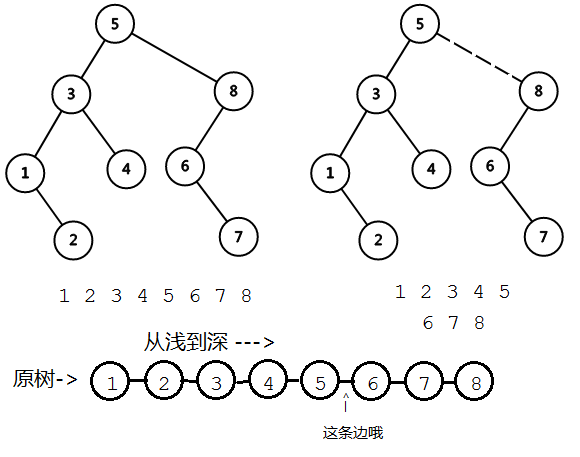## 前言

• 所有的点都包含且仅包含在一条实链中；（也就是一个点也可以成为一条实链）
• 一条实链不包含深度相同的点；（当然了，点的深度是连续变化的，不要问为什么，问就是不知道）
• 我们用一棵Splay树维护一条实链，所以我们维护了一片Splay森林；
• 一棵Splay树的中序遍历代表了这棵Splay树维护的原树的实链从浅到深的点编号；
• 一棵Splay树的根节点可能也有父亲，指向这棵Splay维护的实链的最浅的节点在原树的父亲（也就是Splay中序遍历的第一个点在原树中的父亲），与其他的Splay边不同的是，这个点的父亲的两个子节点均不是这个点（虚边认父不认子）
• 除此以外Splay的形态与原树再无关系（如有错误请指出）

Splay树是一棵BST，所以我们任意进行旋转操作，而保证我们维护的原树信息不被破坏（即旋转不破坏中序遍历）

## LCT操作

struct Node
{
Node *fa, *c;
int v, s;//v:点权 s:子树和自己的xor和
bool t;//翻转标记
};



### update、pushdown、rotate、splay

update别名pushup

inline void update(void)
{
s = v ^ (c ? c->s : 0) ^ (c ? c->s : 0);
//只是这道题的update
}

inline void reverse(void)
{
t ^= 1;
swap(c, c);
}

inline void pushdown(void)
{
if (t)
{
t ^= 1;
if (c)
c->reverse();
if (c)
c->reverse();
}
}


inline bool not_root(void) { return fa && (fa->c == this || fa->c == this); }
inline void rotate()
{
Node *ffa = fa->fa;
bool ty = which();
if (fa->not_root())//这里！！
ffa->c[fa->which()] = this;
fa->c[ty] = c[!ty];
if (fa->c[ty])
fa->c[ty]->fa = fa;
fa->update();
c[!ty] = fa;
fa->fa = this;
fa = ffa;//一棵Splay树的根节点的父亲指向这棵Splay维护的实链的最浅的节点
//在**原树**的父亲（也就是Splay中序遍历的第一个点在**原树**中的父亲）
update();
}


inline void down(void)
{
stack<Node*> st;
st.push(this);
for (Node *i = this; i->not_root(); i = i->fa)
st.push(i->fa);//这样保证Splay根能进入栈
do
st.top()->pushdown();
while (st.pop(), st.empty() == false);
}
inline void splay(void)
{
down();
while (not_root())
{
if (fa->not_root())//记得用新方式
((fa->which() == which()) ? fa : this)->rotate();
rotate(); //双旋啊喂
}
}


### access

（下面的东西会很严格地区分Splay树和原树，我学的时候就是在这方面理解了很久。设计哪种树的会加粗显示）

access：打通原树根节点到指定点的路径（并且指定点下面不再链接实边）

1. 将当前点Splay到根以确保其父亲指向其实是虚边
2. 将当前点的右儿子换成之前的Splay根
3. 你改变了儿子，记得更新...
4. 麻烦你的父亲认你

1. 当你是最开始的点的时候，p=0，相当于切断了与原树更深的点的联系，这时你所在的Splay树维护的实链中，你就是中序遍历最后面一个点；
2. x=x->fa的时候已经记录了你，这时候你的原树父亲也Splay了，并且也让自己成为了她在的实链的最深点，然而她还是你的原树父亲，你们两个人之间只相隔一条虚边，这时你的爸爸认了你，OK你们之间的边就是实边了；
3. 但是你良心发现，爸爸原来认的那个右儿子直接被砍了！你很担心父亲原来认的右儿子，因为那个儿子和你的爸爸之间变成了虚边，而按照定义，这条虚边必须从/一个Splay的根/链接到/Splay对应的实链最浅点/在原树的父亲，于是你就问老猫你自己，这条被“降级的”边有这个性质吗？
（再次强调，一棵Splay维护一条从浅到深的实链）
于是老猫告诉你你告诉你自己，想一想更改儿子前这个Splay的中序遍历，然后想一想删除(而不是替换)这条实边以后原来的Splay的中序遍历和新的Splay的中序遍历。这个我倒是可以画个很简单的图：Node *access(Node *ch = 0)
{
Node *p = 0;//记录上一棵Splay树的根，因为要打成实链，就让当前点认儿子就好了
//再次强调，一棵Splay树只维护从上到下的一条链，所以任意旋转都不会破坏其对于的原树信息
for (Node *x = this; x; p = x, x = x->fa)
{
x->splay();
x->c = p;
x->update();
}
return p;
}


### make_root

inline void make_root() { access()->reverse(); }


inline void make_root()
{
access();
splay();
reverse();
}


### find_root

inline Node *find_root()
{
access();
splay();
Node *r = this;
while (r->c)
r = r->c;
return r;
}


### split

inline void split_to(Node *bottom)
{
bottom->make_root();
access();
splay();
}


inline void link_to(Node *to)
{
make_root();
if (to->find_root() == this)
return;
splay();//这里和其他模板可能不太一样
//是因为我们的make_root不保证当前点在Splay根
fa = to;
}


### cut

inline void cut(Node *another)
{
another->make_root();
if (find_root() !=another)
return;
if (c == 0 || another->fa != this)
return;
//assert(another->c == 0 && another->c == 0);
//这句话亲测不会assert fail
c = another->fa = 0;
update();
}


  B
/ \
A   ...


## （大家都不想看的）代码

#include <cstdio>
#include <algorithm>
#include <cctype>
#include <cassert>

{
//...

using std::min;
using std::swap;

const int N = 100000 + 10;

struct Node
{
Node *fa, *c;
int v, s;
bool t;
inline void update(void) { s = v ^ (c ? c->s : 0) ^ (c ? c->s : 0); }
inline void reverse(void)
{
t ^= 1;
swap(c, c);
}
inline void pushdown(void)
{
if (t)
{
t ^= 1;
if (c)
c->reverse();
if (c)
c->reverse();
}
}
inline bool not_root(void) { return fa && (fa->c == this || fa->c == this); }
inline bool which(void) { return fa->c == this; }
inline void rotate()
{
Node *ffa = fa->fa;
if (fa->not_root()) {
ffa->c[fa->which()] = this;
}
bool ty = which();
fa->c[ty] = c[!ty];
if (fa->c[ty])
fa->c[ty]->fa = fa;
fa->update();
c[!ty] = fa;
fa->fa = this;
fa = ffa;
update();
}
inline void down(void)
{
static Node *st[N];
static int cnt;
cnt = 1;
st = this;
for (Node *i = this; i->not_root(); i = i->fa)
st[++cnt] = i->fa;//DEBUG
for (; cnt; --cnt)
st[cnt]->pushdown();
}
inline void splay(void)
{
down();
while (not_root())
{
if (fa->not_root())
((fa->which() == which()) ? fa : this)->rotate();
rotate(); //DEBUG
}
}
Node *access(Node *ch = 0)
{
Node *p = 0;
for (Node *x = this; x; p = x, x = x->fa)
{
x->splay();
x->c = p;
x->update();
}
return p;
}
inline void make_root()
{
access()->reverse();
}
inline void link_to(Node *to)
{
make_root();
if (to->find_root() == this)
return;
splay();
fa = to;
}
inline void split_to(Node *bottom)
{
bottom->make_root();
access();
splay();
}
inline Node *find_root()
{
access();
splay();
Node *r = this;
while (r->c)
r = r->c;
return r;
}
inline void cut(Node *another)
{
another->make_root();
if (find_root() !=another)
return;
if (c == 0 || another->fa != this)
return;
c = another->fa = 0;
update();
}
}nd[N];

int main(void)
{
int n = read, m = read;
for (int i = 1; i <= n; ++i)
nd[i].v = nd[i].s = read;
while (m--)
{
int opt = read, x = read, y = read;
switch (opt)
{
case 0:
nd[x].split_to(nd + y);
printf("%d\n", nd[x].s);
break;
case 1:
break;
case 2:
nd[x].cut(nd + y);
break;
default:
nd[x].splay();
nd[x].v = y;
nd[x].update();
break;
}
}
}